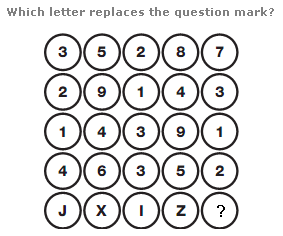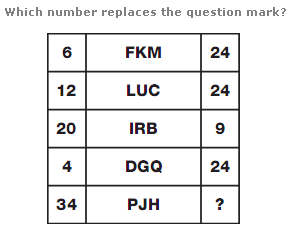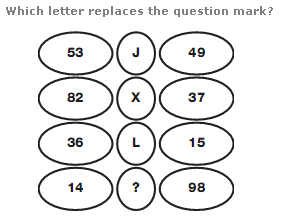# Puzzles - Logical puzzles

### Exercise :: Logical puzzlesAnswer : M Explanation : In each column, add up each number and put the letter with this sum in the bottom circle.

View Answer & ExplanationAnswer : 0 Explanation : In each row, the left hand number equals the total of the even valued letters in the middle box, and the right hand number equals the total of the odd valued letters in the middle box.

View Answer & ExplanationAnswer : C Explanation : In each row, calculate the difference between the 2 numbers in the left hand column, and the 2 numbers in the right hand column. Multiply these differences together to give the numerical value of the letter in the centre.

View Answer & Explanation

#### Current Affairs 2019

Interview Questions and Answers